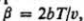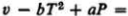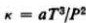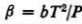### Create an Account

Home / Questions / A hypothetical substance has an isothermal compressibility K a v aft and an expansively ...

# A hypothetical substance has an isothermal compressibility K a v aft and an expansively where a and bare constants a Show that the equation of state is given by constant b If at a pressure

A hypothetical substance has an isothermal compressibility K =a/v aft and an expansivelywhere a and bare constants. {a) Show that the equation of state is given byconstant. {b) If at a pressure P0 and temperature T0, the specific volume is T0, evaluate the constant.

2. A substance has an isothermal compressibilityand an expansivelywhere a and bare constants. Find the equation of state of the substance and the ratio, a/b.

Jun 06 2020 View more View LessSubscribe To Get Solution